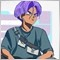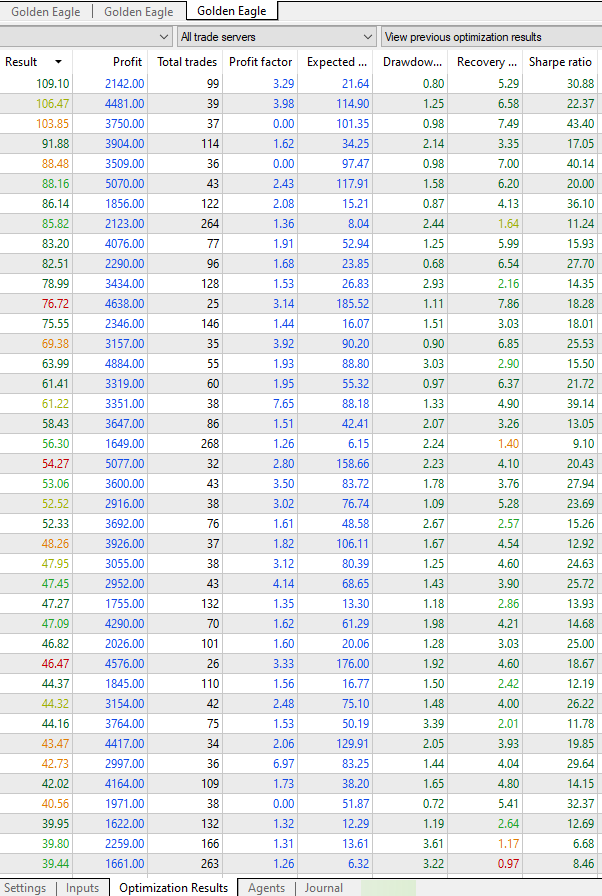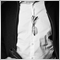# Custom Criteria Coding issues476

Will the following code work?

double profit_factor = TesterStatistics(STAT_PROFIT_FACTOR);

if(STAT_EQUITYDD_PERCENT < 2.5)

if(STAT_PROFIT_FACTOR == 0.0)

{

profit_factor = 500;

}

I'm trying to make it so zero losses that have over a certain number of trades and under a certain DD % will turn that frown upside dow... I mean turn the zero into an integer to plug into the custom return param calculation.

Can I use multiple "If()"s like that in a row? I'm new to coding, so please forgive my ignorance. Should I use the following instead?

if(STAT_EQUITYDD_PERCENT < 2.5 &&  STAT_TRADES > 10 &&  STAT_PROFIT_FACTOR == 0.0)419

Nicholas C Weber:

Will the following code work?

double profit_factor = TesterStatistics(STAT_PROFIT_FACTOR);

if(STAT_EQUITYDD_PERCENT < 2.5)

if(STAT_PROFIT_FACTOR == 0.0)

{

profit_factor = 500;

}

I'm trying to make it so zero losses that have over a certain number of trades and under a certain DD % will turn that frown upside dow... I mean turn the zero into an integer to plug into the custom return param calculation.

Can I use multiple "If()"s like that in a row? I'm new to coding, so please forgive my ignorance. Should I use the following instead?

if(STAT_EQUITYDD_PERCENT < 2.5 &&  STAT_TRADES > 10 &&  STAT_PROFIT_FACTOR == 0.0)

I did not fully understand your explanation but the way you have written the if's will not work or compile.

I suspect you want to "and" the conditions together so when all three are true you set profit_factor

It would look like this:

```   double profit_factor = TesterStatistics(STAT_PROFIT_FACTOR);

if(
(STAT_EQUITYDD_PERCENT < 2.5)
&& (STAT_PROFIT_FACTOR == 0.0)
)

{

profit_factor = 500;

}
```

Also please use Alt+s or the "code" button on the tool bar when pasting code476

R4tna C #:

I did not fully understand your explanation but the way you have written the if's will not work or compile.

I suspect you want to "and" the conditions together so when all three are true you set profit_factor

It would look like this:

Also please use Alt+s or the "code" button on the tool bar when pasting code

` if(STAT_EQUITYDD_PERCENT < 2.5 &&  STAT_TRADES > 10 &&  STAT_PROFIT_FACTOR == 0.0)`

That has compiled and the optimization results are showing favorable. I will try your

```   double profit_factor = TesterStatistics(STAT_PROFIT_FACTOR);

if(
(STAT_EQUITYDD_PERCENT < 2.5)
&& (STAT_PROFIT_FACTOR == 0.0)
)

{

profit_factor = 500;

}```

code next and see if there's a difference.

I added a screenshot to show the results of the code as is before changing to your code. I greatly appreciate your reply! Thanks.As you can see, a result with a profit factor of 0, and meeting the other 2 criteria, has scored higher up, where-as before it would appear way further down. I think line breaks aren't necessary(from what I read on an article talking about javascript.. not MQL5 specifically), just semicolons and parenthesis. Though I see that there are no parenthesis on the opposite ends of the && code I am currently using. Should it not have worked, otherwise?

Files:476

Is there a way to change from 2 separate optimization custom criteria in a single EA? I guess you would set a constant for true/false to choose between 2 criterion calculations?419

Nicholas C Weber #:

That has compiled and the optimization results are showing favorable. I will try your

code next and see if there's a difference.

Yes I noticed you had put

` if(STAT_EQUITYDD_PERCENT < 2.5 &&  STAT_TRADES > 10 &&  STAT_PROFIT_FACTOR == 0.0)`

This works also but I recommend using brackets, many people don't but I feel it is better programming practice and helps avoid subtle errors.

Sometimes it can be better to go further, like below, as this allows you to put a breakpoint on the if statement and see what each of the terms evaluates to - helpful when analyzing complex logic with many aspects

```   double profit_factor = TesterStatistics(STAT_PROFIT_FACTOR);
bool  bool_STAT_EQUITYDD_PERCENT = (STAT_EQUITYDD_PERCENT < 2.5);
bool  bool_STAT_PROFIT_FACTOR = (STAT_PROFIT_FACTOR == 0.0);

if(
bool_STAT_EQUITYDD_PERCENT
&& bool_STAT_PROFIT_FACTOR
)

{

profit_factor = 500;

}

```2687

Ähm...

People, these are enumerations.

You cannot use them like that.

` if(STAT_EQUITYDD_PERCENT < 2.5 &&  STAT_TRADES > 10 &&  STAT_PROFIT_FACTOR == 0.0)`

` if(TesterStatistics(STAT_EQUITYDD_PERCENT) < 2.5)`476

So, would this work?
```  double  balance = TesterStatistics(STAT_PROFIT);
if(balance > 0.0)
{
balance = (balance);
}
else if(balance < 0.0)
{
balance = (-1.0);
}
else if(balance == 0.0)
{
balance = (0.0);
}

double equity_dd = TesterStatistics(STAT_EQUITY_DD);

double balance_dd = TesterStatistics(STAT_BALANCE_DD);

double profit_factor = TesterStatistics(STAT_PROFIT_FACTOR);
if(profit_factor > 0.0)
{
profit_factor = (profit_factor / 10);
}
else if(profit_factor == 0.0
&& ((equity_dd + balance_dd) / 2) < 2.5
&& balance > 0.0)
{
profit_factor = (500);
}```476

There was an error, I fixed it and updated my reply..419

Nicholas C Weber #
So, would this work?

In terms of whether it will work, there are 2 considerations

1. first will it compile/run

2. does it satisfy the intended algo

Q2. is not something I can answer, but for Q1 there were compilation errors - here is an adjusted version with the corrections highlighted with comments.

I recommend using the NormalizeDouble function when comparing doubles - often there can be a mismatch so you have to enforce accuracy.

```   double  balance = TesterStatistics(STAT_PROFIT);
if(NormalizeDouble(balance, 1) > NormalizeDouble(0.0, 1) ) //Recommendation (example only of 1 decimal place) - apply to all double comparisons
{
balance = (balance);
}
else
if(balance < 0.0)
{
balance = (-1.0);
}
else
if(balance == 0.0)
{
balance = (0.0);
}

double equity_dd = TesterStatistics(STAT_EQUITY_DD);

double balance_dd = TesterStatistics(STAT_BALANCE_DD);

double profit_factor = TesterStatistics(STAT_PROFIT_FACTOR);
int    profit_factor_integer = (int) profit_factor;    //Addition 1
if(profit_factor_integer > 0.0)
{
profit_factor = (profit_factor / 10);
}
else
(profit_factor == 0.0)
&& (((equity_dd + balance_dd) / 2) < 2.5)
&& (balance > 0.0)
{
profit_factor = (500);
}
```476

R4tna C #:

In terms of whether it will work, there are 2 considerations

1. first will it compile/run

2. does it satisfy the intended algo

Q2. is not something I can answer, but for Q1 there were compilation errors - here is an adjusted version with the corrections highlighted with comments.

I recommend using the NormalizeDouble function when comparing doubles - often there can be a mismatch so you have to enforce accuracy.

Yeah, I left in profit_factor_integer because I thought I was getting slick with integer and zero versions, but scratched it and forgot to edit that before pasting. So sloppy. My apologies, mon capitan.

Here is my complete code, to check. I hope I got win_ratio  set up correctly.

```double OnTester()
{
double  param = 0.0;

//  Balance Max + Min Drawdown + Profit Trades Number + Profit Factor + Recovery Factor + Win Ratio:

double  balance = TesterStatistics(STAT_PROFIT);
if(balance > 0.0)
{
balance = (balance);
}
else
if(balance < 0.0)
{
balance = (-1.0);
}
else
if(balance == 0.0)
{
balance = (0.0);
}

double  min_dd = TesterStatistics((STAT_EQUITY_DD && STAT_BALANCE_DD) / 2);
if(min_dd > 0.0)
{
min_dd = (1.0 / min_dd);
}
else
if(min_dd == 0.0)
{
min_dd = (1);
}

{
}

double equity_dd = TesterStatistics(STAT_EQUITY_DD);

double balance_dd = TesterStatistics(STAT_BALANCE_DD);

double profit_factor = TesterStatistics(STAT_PROFIT_FACTOR);
if(profit_factor > 0.0)
{
profit_factor = (profit_factor / 10);
}
else
if(
(profit_factor == 0.0)
&& (((equity_dd + balance_dd) / 2) < 2.5)
&& (balance > 0.0)
)
{
profit_factor = (500);
}

double recovery_factor = TesterStatistics(STAT_RECOVERY_FACTOR);

if(win_ratio > 1.4)
{
win_ratio = ( win_ratio / 10);
}
else
if(win_ratio < 1.5)
{
win_ratio = (0);
}
else
if(win_ratio > 25)
{
win_ratio = (win_ratio);
}
else
if(win_ratio > 50)
{
win_ratio = (win_ratio * 2);
}

param = (balance * min_dd * (profit_trades * win_ratio) * (recovery_factor + profit_factor / 2) / 100);

return(param);
}```

I'm trying to make win_ratio Profit trades number divided by loss trades number. I understand profit factor being total profit amount divided by total loss amount, and I needed a win ratio in the calculations to prevent a bunch of small losses sprinkled everywhere. those results would be a fraction and win ratios over 1.0 would not reduce the custom criterion result number, otherwise. ..And prioritizing the high win rate by multiplying it's result.419

Nicholas C Weber #:

Yeah, I left in profit_factor_integer because I thought I was getting slick with integer and zero versions, but scratched it and forgot to edit that before pasting. So sloppy. My apologies, mon capitan.

Here is my complete code, to check. I hope I got win_ratio  set up correctly.

I'm trying to make win_ratio Profit trades number divided by loss trades number. I understand profit factor being total profit amount divided by total loss amount, and I needed a win ratio in the calculations to prevent a bunch of small losses sprinkled everywhere. those results would be a fraction and win ratios over 1.0 would not reduce the custom criterion result number, otherwise.

Sure - please do follow the NormalizeDouble() recommendation, may not be apparent at first but I have hit subtle bugs when it was omitted.

Good luck with you trading and ongoing coding